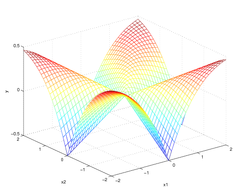Print page

# VisualizationsIn this page we present some first (nonconvex) visualizations of the directed subdifferential:

3d examples     • 2d examples     • anaglyphs (3d stereo)     • SIRDS (autostereograms)

Instead looking at the small pictures (mostly 160x120 pixels), click on the links to see the picture in full-size (mostly 640x480 pixels).

Due to the idea of Alexander Rubinov in 2000 (at the conference in Samos, Greece) to subtract subdifferentials of convex analysis to get a nonconvex subdifferential of a DC function, the visualization of the directed subdifferential (formally, an element of the Banach space of directed sets) is named Rubinov subdifferential.

## 3D Examples

### f(x) = g(x) - r*h(x) = (|x1| + |x2| + |x3|) - r*sqrt(x12 + x22 + x32)

Since the subdifferential of convex analysis of the 1-norm function g(x) in x=0 equals the unit cube [-1,1]3 and the subdifferential of the square root function h(x) in x=0 equals the unit ball B1(0), we have to subtract the unit cube minus the ball with the origin as center and the radius r to calculate the directed subdifferential of f(x) in x=0.

## 2D Examples

### f(x) = g(x) - r*h(x) = (|x1| + |x2|) - r*sqrt(x12 + x22)

The situation is similar to the 3d case, we subtract the unit square [-1,1]2 minus r times the unit circle B1(0) to calculate the directed subdifferential of f(x) in x=0.

## 3D Stereo Images (Anaglyphs)

You need red/cyan glasses to view the 3d stereo pictures (anaglyphs).

Many thanks to Fabian Kißler who wrote the software and created the pictures.

### f(x) = g(x) - h(x) = sqrt(x12 + x22 + x32) - (|x2| + |x3|)

Since the subdifferential of convex analysis of the function h(x) in x=0 equals the 3d unit square {0} x [-1,1]2 and the subdifferential of the square root function h(x) in x=0 equals the unit ball B1(0), we have to subtract the unit ball minus the unit square {0} x [-1,1]2 to calculate the directed subdifferential of f(x) in x=0.

## 3D Stereo Images (SIRDS)

You need quite some training to view the 3d stereo pictures in the SIRDS format (random dot autostereogram). The animated SIRDS picture always shows the same 3d picture, the animation with another random pixel field only helps to easier view the SIRDS picture. Keep in mind, that SIRDS pictures of such complicated nonconvex figures usually result in a rather poor 3d impression.

You should try to look at a plane behind the picture for a longer time, do not try to focus a particular pixel of the images. If after a while a spatial impression starts to evolve, do not focus on a particular part of the image (keep on staring behind the picture or at least at corners of the SIRDS picture). The distance to the monitor is important, try to have a distance around 30 cm. Sometimes it helps to vary gradually the distance and be patient ... . If you succeed your first picture, you will much easier see all the rest ... . If you have problems, download the SIRDS picture and look at it in full screen mode (same distance).

Many thanks to Fabian Kißler who wrote the software and created the pictures.

### f(x) = g(x) - h(x) = sqrt(x12 + x22 + x32) - (|x2| + |x3|)

For training to view at SIRDS you can view the following pictures showing just a unit ball (the subdifferential of the Euclidean norm at x=0):

responsible for the content: Lars Grüne